# Row reduction and echelon forms

### Row reduction and echelon forms

#### Lessons

A non-zero row is a row that has at least one entry that is not zero.

The leading entry of a row is the leftmost non-zero entry in a non-zero row.

A rectangular matrix is in echelon form if it has the three properties:

1. All non-zero rows are above any rows of all zeros.
2. Each leading entry of a row is in a column to right of the leading entry of the row above it.
3. All entries in a column below a leading entry are zeros.

If the rectangular matrix satisfies 2 more additional properties, then it is in reduced echelon form:

1. The leading entry in each non-zero row is 1.
2. Each leading 1 is the only non-zero entry in its column.

Essentially the difference between the two forms is that the reduced echelon form has 1 as a leading entry, and the column of the leading entry has 0's below and above.

The pivot position is just the leading entries of the echelon form matrix.

The pivot column is the column of the pivot position.

Here are the steps for the row reduction algorithm:

1. Look for the leftmost non-zero column. This is our pivot column.
2. Find a non-zero entry in the pivot column. This is our pivot position. It should be at the very top of the pivot column.
3. Use matrix row operations to make all the entries below the pivot 0.
4. Ignore the row with the pivot. Repeat Step 1-3 again and again until you can't anymore.
5. Find your rightmost pivot. Make it 1. Then make all the entries above it 0.
• Introduction
Row Reduction and Echelon Form Overview:
a)
Echelon Matrix vs. Reduced Echelon Matrix
• 3 properties for echelon form
• Addition 2 properties for reduced echelon form
• What is the difference between them?

b)
Pivot Position and Pivot Column
• pivot position = leading entry
• pivot column = column of pivot position

c)
The Row Reduction Algorithm
• The 5 steps of the algorithm
• Making sure it is in reduced echelon form

d)
Solutions of Linear Systems
• Reduced echelon form of augmented matrix
• Basic variables and free variables
• Writing out the solutions

• 1.
Difference between Echelon Form and Reduced Echelon Form
Label whether the following matrices are in echelon form or reduced echelon form:
a)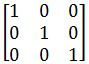b)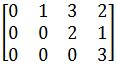c)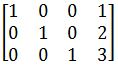d)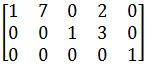• 2.
Learning the Row Reduction Algorithm
Row reduce the matrices to reduced echelon form. Circle the pivot positions in the final and original matrix, and list the pivot columns from the original matrix in part b:
a)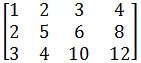b)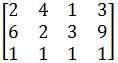• 3.
Finding the General Solution of a Matrix
Find the general solution of the following matrices:
a)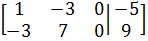b)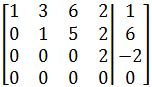• 4.
Linear Systems with Unknown Constants
Choose values of a and b where the system has infinitely many solutions, and no solutions:
a)
$x_1+ax_2=4$
$2x_1+4x_2=b$

b)
$3x_1-x_2=6$
$x_1+ax_2=b$